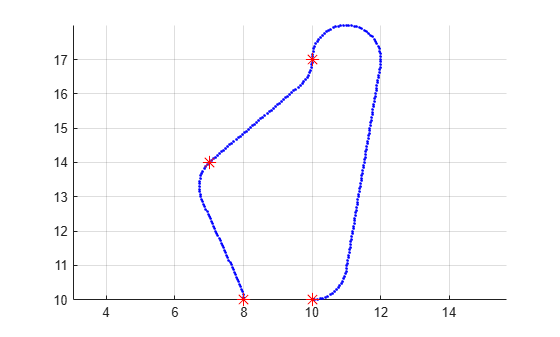# interpolate

Interpolate path based on propagator step size

## Syntax

``interpolate(pathObj)``

## Description

example

````interpolate(pathObj)` evaluates the path based on the `ControlStepSize` property of `pathObj`, and adds all intermediate points to the path.```

## Examples

collapse all

Create a `navPath` object based on multiple waypoints in a Dubins space.

`dubinsSpace = stateSpaceDubins([0 25; 0 25; -pi pi])`
```dubinsSpace = stateSpaceDubins with properties: SE2 Properties Name: 'SE2 Dubins' StateBounds: [3x2 double] NumStateVariables: 3 Dubins Vehicle Properties MinTurningRadius: 1 ```
`pathobj = navPath(dubinsSpace)`
```pathobj = navPath with properties: StateSpace: [1x1 stateSpaceDubins] States: [0x3 double] NumStates: 0 ```
```waypoints = [8 10 pi/2; 7 14 pi/4; 10 17 pi/2; 10 10 -pi]; append(pathobj,waypoints)```

Interpolate that path so that it contains exactly 250 points.

`interpolate(pathobj,250)`

Visualize the interpolated path and the original waypoints.

```figure grid on axis equal hold on plot(pathobj.States(:,1),pathobj.States(:,2),".b") plot(waypoints(:,1),waypoints(:,2),"*r","MarkerSize",10)```Calculate length of path.

```len = pathLength(pathobj); disp("Path length = " + num2str(len))```
```Path length = 19.4722 ```

## Input Arguments

collapse all

Control path, specified as a `navPathControl` object.

Data Types: `double`

## Version History

Introduced in R2021b